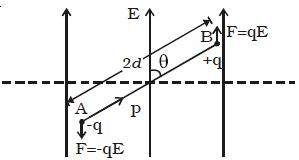# Explain Electric Potential Energy of an Electric Dipole in an Electric Field

The electric potential energy of an electric dipole in an electrostatic field is the work done in rotating the dipole to the desired position in the field.

When an electric dipole of dipole moment p is at an angle θ with the electric field E, the torque on the dipole is

τ = pE sin θWork done in rotating the dipole through dθ,

dw = τ.dθ = pE sinθ.dθ

The total work done in rotating the dipole through an angle θ is W = ∫dw

W = pE ∫sinθ.dθ = –pE cos θ

This work done is the potential energy (U) of the dipole.

so, U = – pE cos θ

When the dipole is aligned parallel to the field, θ = 00

so, U = –pE

This shows that the dipole has a minimum potential energy when it is aligned with the field. A dipole in the electric field experiences a torque (τ = p * E) which tends to align the dipole in the field direction, dissipating its potential energy in the form of heat to the surroundings.